# Chapter 7 Optimization Content Introduction One dimensional unconstrained

• Slides: 25Chapter 7 OptimizationContent • Introduction • One dimensional unconstrained • Multidimensional unconstrained • Example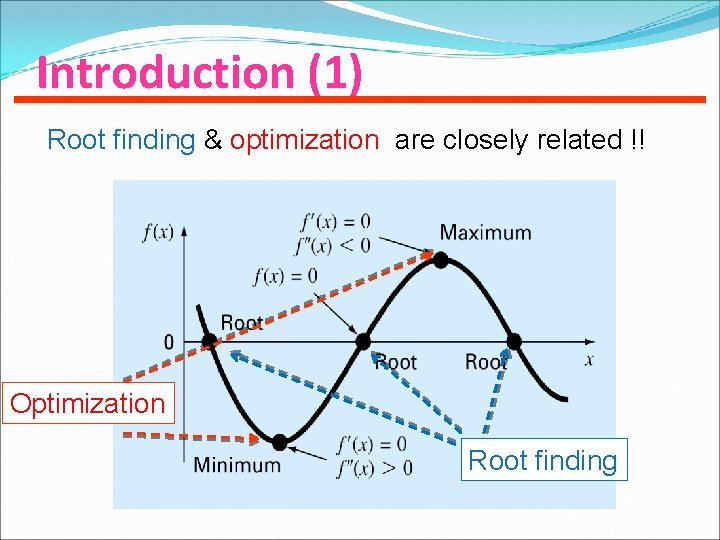Introduction (1) Root finding & optimization are closely related !! Optimization Root finding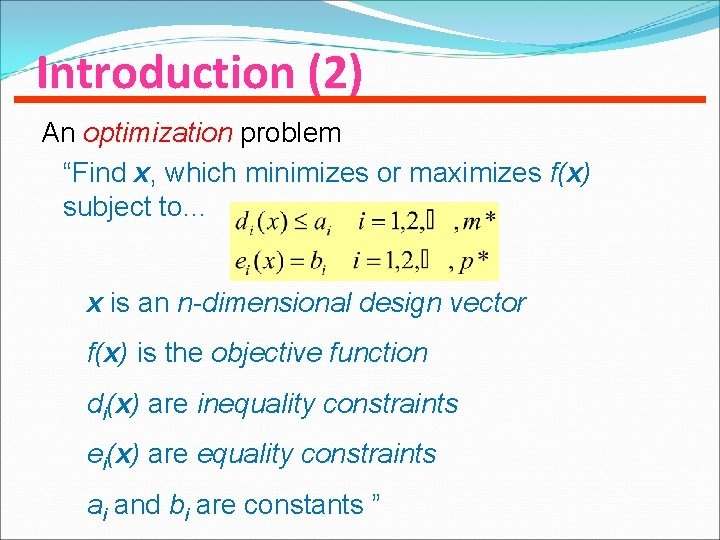Introduction (2) An optimization problem “Find x, which minimizes or maximizes f(x) subject to… x is an n-dimensional design vector f(x) is the objective function di(x) are inequality constraints ei(x) are equality constraints ai and bi are constants ”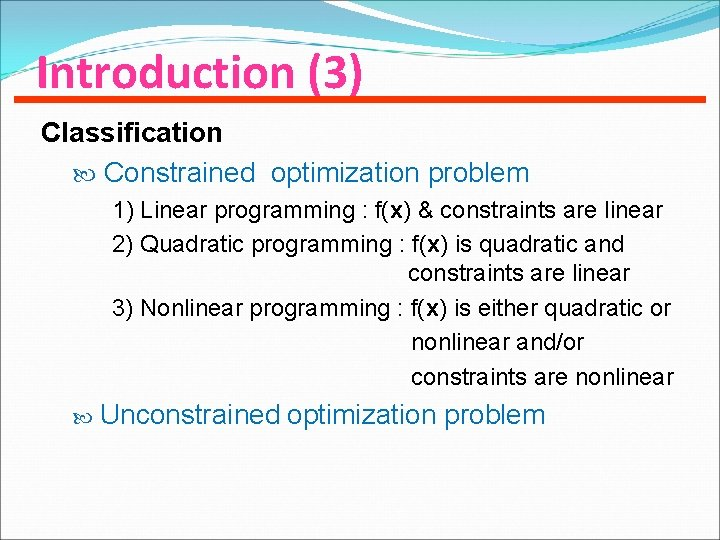Introduction (3) Classification Constrained optimization problem 1) Linear programming : f(x) & constraints are linear 2) Quadratic programming : f(x) is quadratic and constraints are linear 3) Nonlinear programming : f(x) is either quadratic or nonlinear and/or constraints are nonlinear Unconstrained optimization problemOne-dimensional (1) interested in finding the absolute highest or lowest value of a function. Multimodal function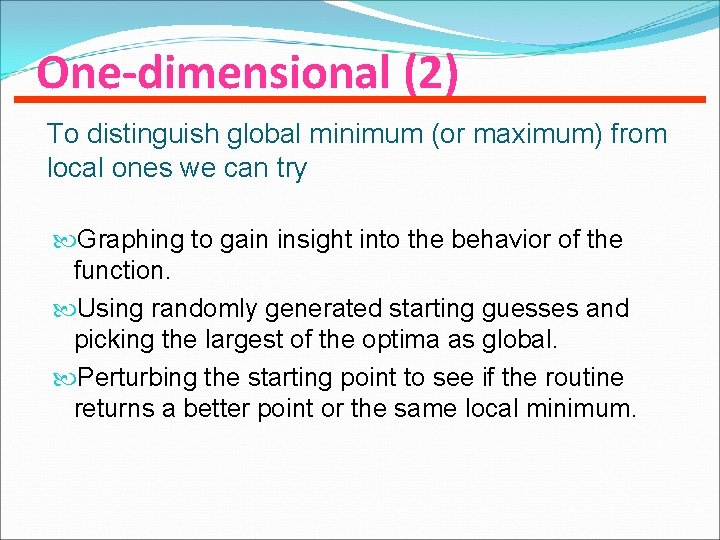One-dimensional (2) To distinguish global minimum (or maximum) from local ones we can try Graphing to gain insight into the behavior of the function. Using randomly generated starting guesses and picking the largest of the optima as global. Perturbing the starting point to see if the routine returns a better point or the same local minimum.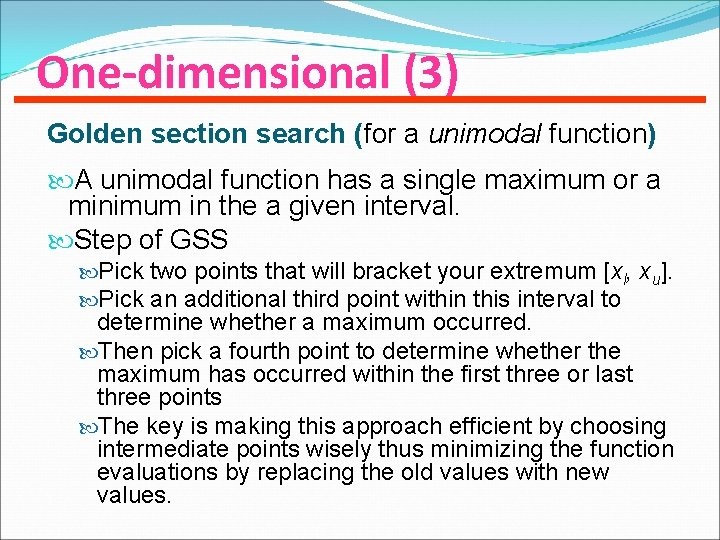One-dimensional (3) Golden section search (for a unimodal function) A unimodal function has a single maximum or a minimum in the a given interval. Step of GSS Pick two points that will bracket your extremum [xl, xu]. Pick an additional third point within this interval to determine whether a maximum occurred. Then pick a fourth point to determine whether the maximum has occurred within the first three or last three points The key is making this approach efficient by choosing intermediate points wisely thus minimizing the function evaluations by replacing the old values with new values.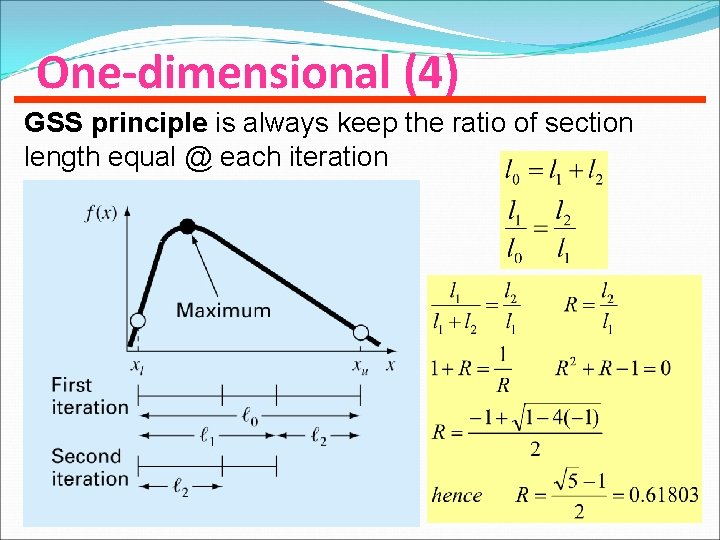One-dimensional (4) GSS principle is always keep the ratio of section length equal @ each iterationOne-dimensional (5) Step I: Starts with two initial guesses (xl , xu) Step II: Calculate points x 1, x 2 according to the golden ratio where Step III: Evaluate function at x 1 and x 2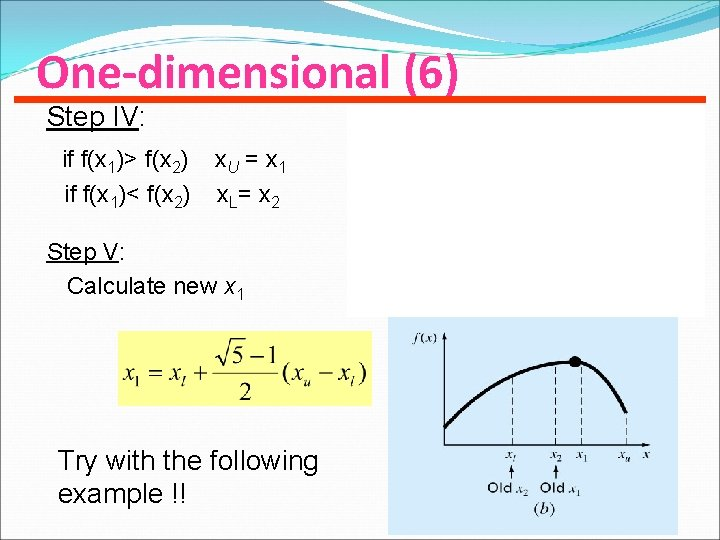One-dimensional (6) Step IV: if f(x 1)> f(x 2) if f(x 1)< f(x 2) x. U = x 1 x L= x 2 Step V: Calculate new x 1 Try with the following example !! x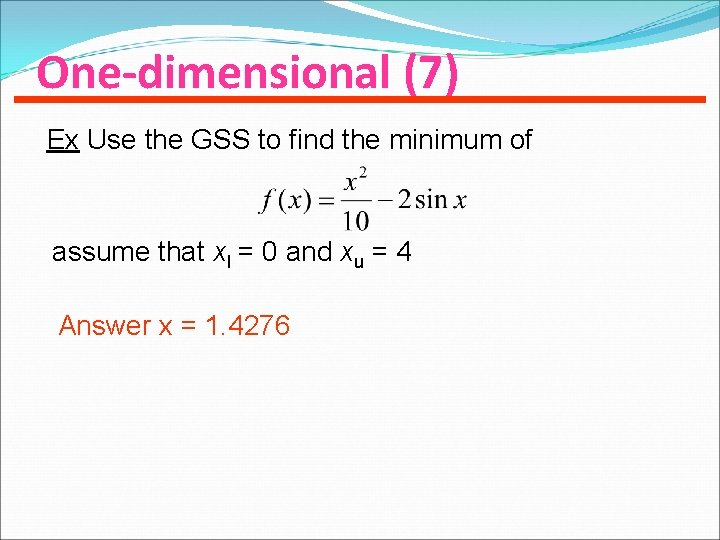One-dimensional (7) Ex Use the GSS to find the minimum of assume that xl = 0 and xu = 4 Answer x = 1. 4276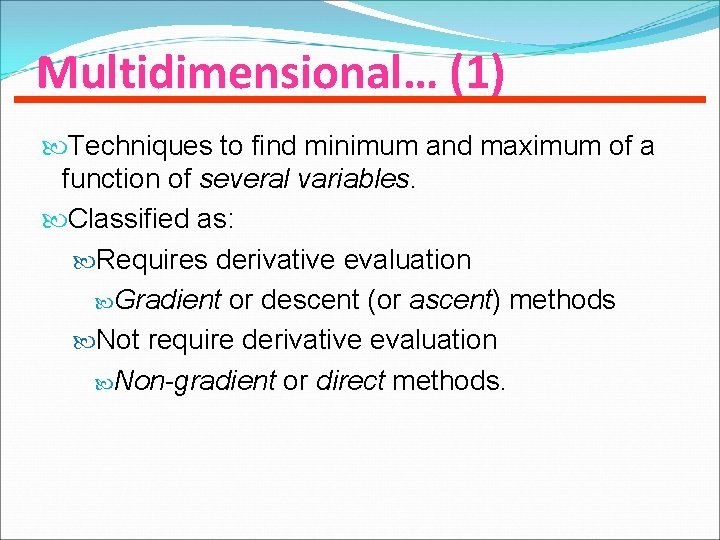Multidimensional… (1) Techniques to find minimum and maximum of a function of several variables. Classified as: Requires derivative evaluation Gradient or descent (or ascent) methods Not require derivative evaluation Non-gradient or direct methods.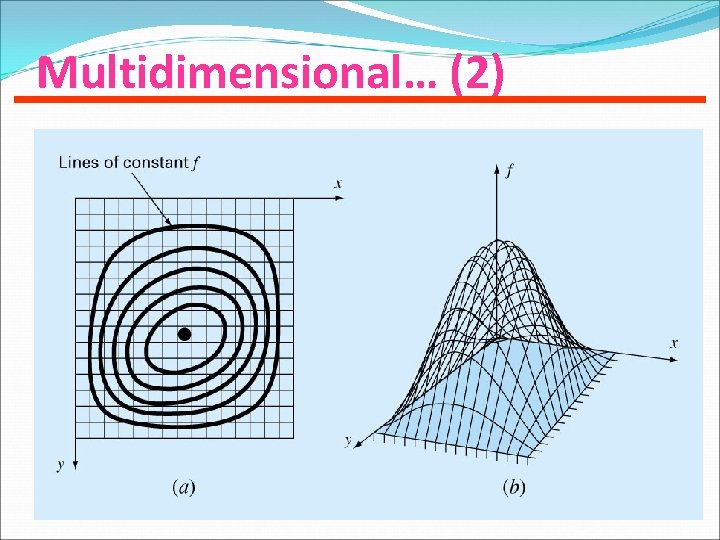Multidimensional… (2)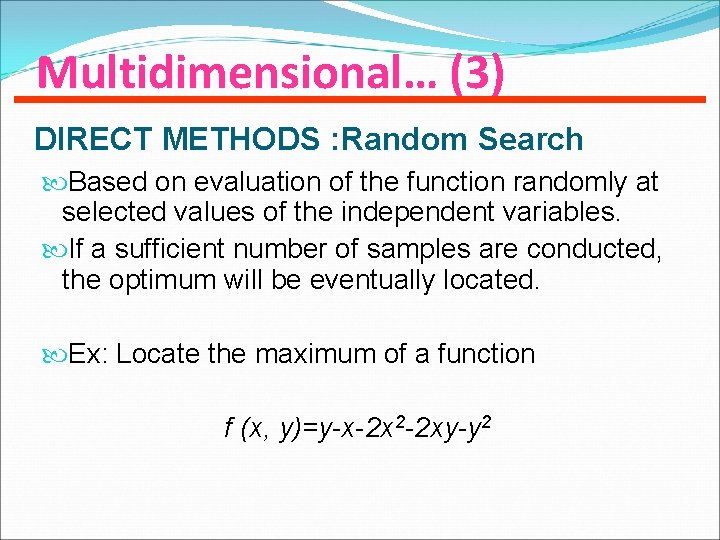Multidimensional… (3) DIRECT METHODS : Random Search Based on evaluation of the function randomly at selected values of the independent variables. If a sufficient number of samples are conducted, the optimum will be eventually located. Ex: Locate the maximum of a function f (x, y)=y-x-2 x 2 -2 xy-y 2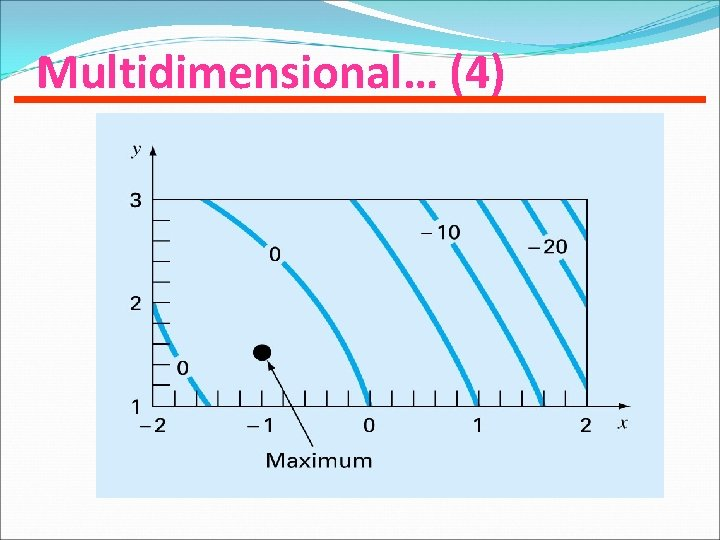Multidimensional… (4)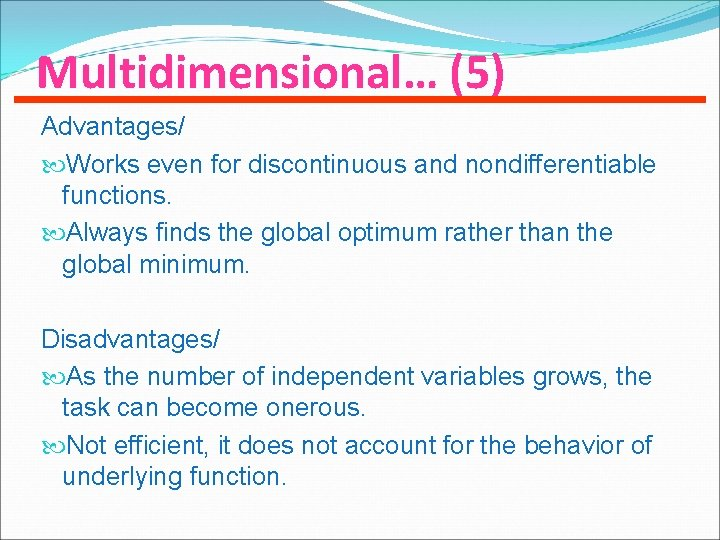Multidimensional… (5) Advantages/ Works even for discontinuous and nondifferentiable functions. Always finds the global optimum rather than the global minimum. Disadvantages/ As the number of independent variables grows, the task can become onerous. Not efficient, it does not account for the behavior of underlying function.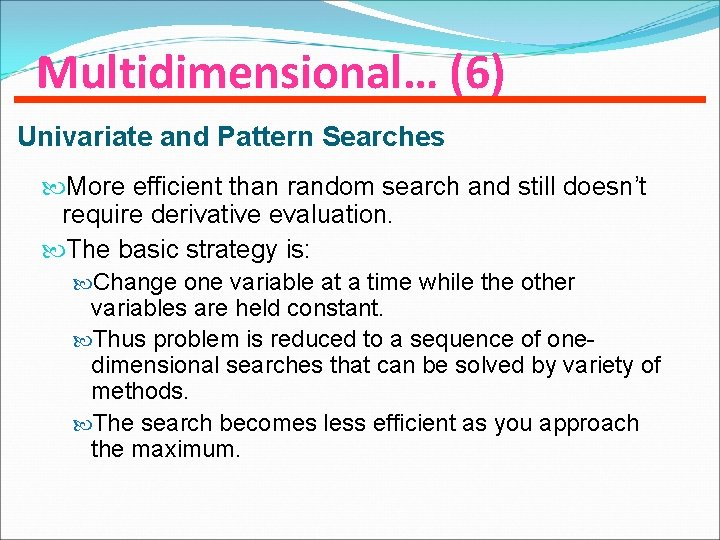Multidimensional… (6) Univariate and Pattern Searches More efficient than random search and still doesn’t require derivative evaluation. The basic strategy is: Change one variable at a time while the other variables are held constant. Thus problem is reduced to a sequence of onedimensional searches that can be solved by variety of methods. The search becomes less efficient as you approach the maximum.Multidimensional… (7) Univariate and Pattern SearchesMultidimensional… (8) Gradient method If f(x, y) is a two dimensional function, the gradient vector tells us What direction is the steepest ascend? How much we will gain by taking that step? Directional derivative of f(x, y) at point x=a and y=bMultidimensional… (9) Gradient method (cont’d) • For n dimensionsMultidimensional… (10) Steepest ascent method Use the gradient vector to change x Says that the we should change with along h-axis The gradient vector represents a direction of maximum rate of increase for the function f(x) at x.Multidimensional… (11) Example Minimize the function where x = 0. 6, y = 4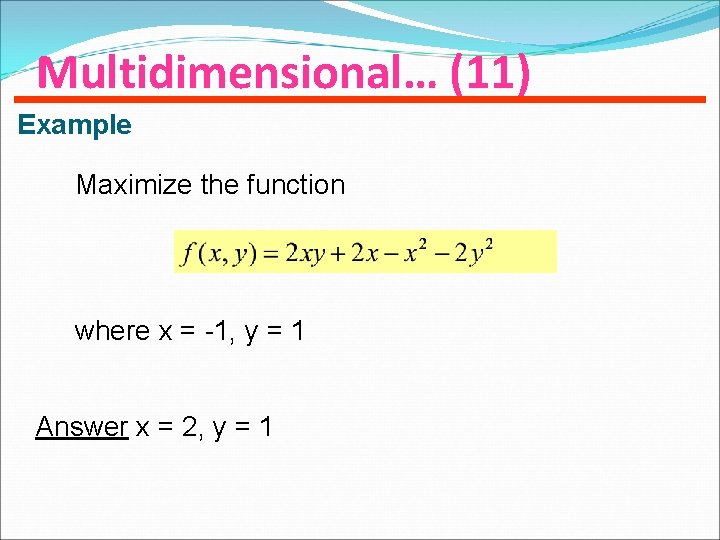Multidimensional… (11) Example Maximize the function where x = -1, y = 1 Answer x = 2, y = 1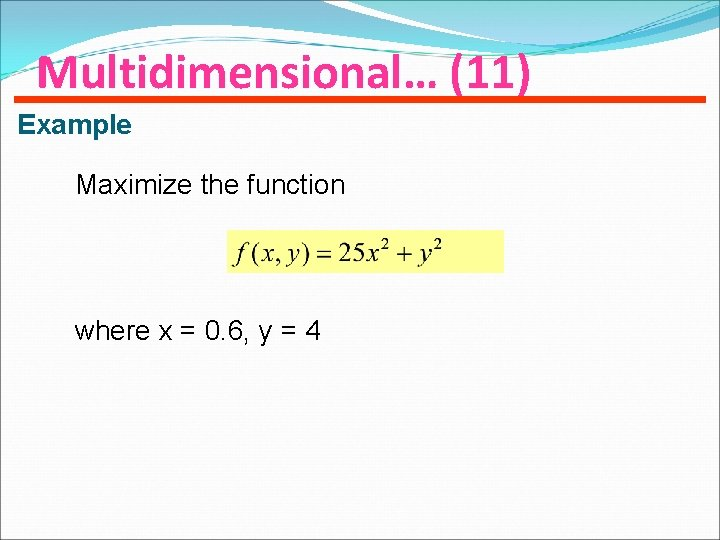Multidimensional… (11) Example Maximize the function where x = 0. 6, y = 4